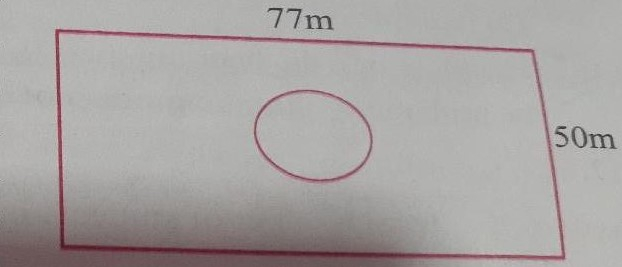"
">

# Length and breadth of a rectangular garden are $77 \mathrm{~m}$ and $50 \mathrm{~m}$. There is a circular lake in the garden having diameter $14 \mathrm{~m}$. Due to wind, a towel from a terrace on a nearby building fell into the garden. Then find the probability of the event that it fell in the lake."

Given:

Length of the rectangular garden$=77\ m$.

Breadth of the rectangular garden$=50\ m$.

Diameter of the lake$=14\ m$.

To do:

We have to find the probability of the event that the towel fell in the lake.

Solution:

The probability of the event that the towel fell in the lake$=$Area of the lake$\div$Area of the rectangular garden.

Radius of the lake$=\frac{14}{2}\ m=7\ m$.

Area of the lake$=\pi (7)^2=\frac{22}{7}\times7\times7=154\ m^2$.

Area of the garden$=77\times50\ m^2=3850\ m^2$

Therefore,

The probability of the event that the towel fell in the lake$=\frac{154}{3850}=\frac{1}{25}$.

Updated on: 10-Oct-2022

249 Views